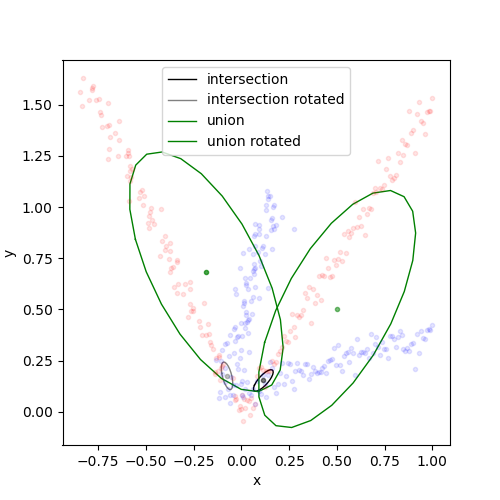﻿ RCFS packages = ['numpy','matplotlib']
def print(x): display(x, target='output') import numpy as np import matplotlib.pyplot as plt from js import document, console from pyodide.ffi import create_proxy def sqrtm(a): # Computing diagonalization evalues, evectors = np.linalg.eig(a) # Ensuring square root matrix exists # assert (evalues >= 0).all() return evectors @ np.diag(np.sqrt(evalues)) @ np.linalg.inv(evectors) def plot_gaussian(mu, sigma, ax, dim=None, color='r', alpha=0.5, lw=1, markersize=6, **kwargs): nb_segm=24 mu, sigma = np.array(mu), np.array(sigma) t = np.linspace(-np.pi, np.pi, nb_segm) R = np.real(sqrtm(1.0 * sigma)) points = np.einsum('ij,ja->ia', R, np.array([np.cos(t), np.sin(t)])) + mu[:, None] center, = ax.plot(mu, mu, '.', color=color, alpha=alpha) # Mean line, = ax.plot(points, points, color=color, linewidth=lw, markersize=markersize, **kwargs) # Contour return center, line def condition_gaussian(x1, slice1, slice2, mu, sigma): mu1 = mu[slice1] mu2 = mu[slice2] sigma11 = sigma[slice1, slice1] sigma22 = sigma[slice2, slice2] sigma12 = sigma[slice1, slice2] sigma11_inv = np.linalg.inv(sigma11) mu_C = mu2 + sigma12 @ sigma11_inv @ (x1 - mu1) sigma_C = sigma22 - sigma12 @ sigma11_inv @ sigma12 return mu_C, sigma_C

# Exercise 3Gaussian Distributions

Multivariate Gaussian distributions have many computationally favorable properties that have been used extensively in robotics. In this exercise, we focus on some operations with Gaussian densities that result in another Gaussian density, namely, linear combination and product of Gaussians. We will see how one can use these operations in regression problems.

##### 1. Multivariate Gaussian Distribution

Fill in the code below to implement linear_combination and product_gaussians.

def gaussian_nD(x, mu, sigma): D = x.shape cons = ((2*np.pi)**(-D/2)) * (np.linalg.det(sigma)**(-0.5)) return cons*np.exp(-0.5*(x-mu).dot(np.linalg.inv(sigma))@ (x-mu)) def linear_combination(A1, A2, c, mu1, mu2, sigma1, sigma2): mu_L = np.zeros(2) # Implement here sigma_L = np.eye(2) # Implement here return mu_L, sigma_L def product_gaussians(mu1, mu2, sigma1, sigma2): sigma1_inv = np.linalg.inv(sigma1) sigma2_inv = np.linalg.inv(sigma2) sigma_P = np.eye(2)# Implement here mu_P = np.zeros(2) # Implement here c = 0. # Implement here return mu_P, sigma_P, c
def gaussian_nD(x, mu, sigma): D = x.shape cons = ((2*np.pi)**(-D/2)) * (np.linalg.det(sigma)**(-0.5)) return cons*np.exp(-0.5*(x-mu).dot(np.linalg.inv(sigma))@ (x-mu)) def linear_combination(A1, A2, c, mu1, mu2, sigma1, sigma2): mu_L = A1 @ mu1 + A2 @ mu2 + c sigma_L = A1 @ sigma1 @ A1.T + A2 @ sigma2 @ A2.T return mu_L, sigma_L def product_gaussians(mu1, mu2, sigma1, sigma2): sigma1_inv = np.linalg.inv(sigma1) sigma2_inv = np.linalg.inv(sigma2) sigma_P = np.linalg.inv(sigma1_inv + sigma2_inv) mu_P = sigma_P @ (sigma1_inv @ mu1 + sigma2_inv @ mu2) c = gaussian_nD(mu1, mu2, sigma1 + sigma2) return mu_P, sigma_P, c

You have access to a plotting function called plot_gaussian(mu, sigma, ax, dim=None, color='r', alpha=0.5, lw=1, markersize=6, **kwargs). You need to give mu : ndarray(N,) , sigma : ndarray(N,N)  and ax : subplot object to the function (you can also give other arguments that matplotlib accepts).

Implement product_gaussians in the code above and run the code below to test your answer. You can verify your answer by clicking on the button below the code.

mu1 = np.zeros(2) mu2 = np.ones(2) sigma1 = np.diag([.5,.1]) sigma2 = np.diag([1,1]) mu_P, sigma_P, c = product_gaussians(mu1, mu2, sigma1, sigma2) plt.close('all') fig,ax = plt.subplots(figsize=(5,5)) plot_gaussian(mu1, sigma1, ax, color='r', label='first Gaussian') plot_gaussian(mu2, sigma2, ax, color='b', label='second Gaussian') plot_gaussian(mu_P, sigma_P, ax, color='k', label='product of Gaussians' ) ax.legend() ax.axis('off') ax.set_aspect('equal') fig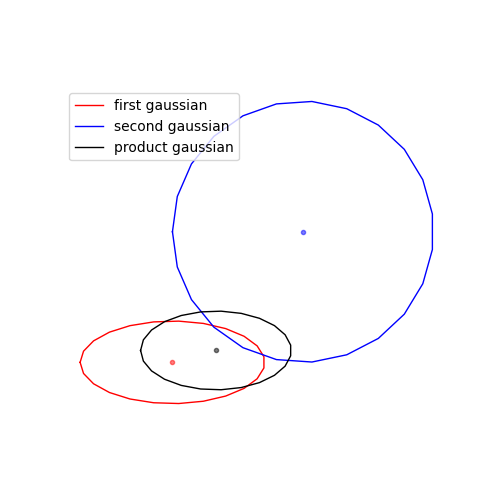Implement linear_combination in the code above and run the code below to test your answer. You can verify your answer by clicking on the button below the code (the plot in the answer is generated with c=0).

mu1 = np.zeros(2) mu2 = np.ones(2) sigma1 = np.diag([1,1]) sigma2 = np.diag([1,1]) A1 = np.diag([1., 1]) A2 = np.diag([1., 1]) c = np.random.randn(2) mu_L, sigma_L = linear_combination(A1, A2, c, mu1, mu2, sigma1, sigma2) plt.close('all') fig,ax = plt.subplots(figsize=(5,5)) plot_gaussian(mu1, sigma1, ax, color='r', label='first Gaussian') plot_gaussian(mu2, sigma2, ax, color='b', label='second Gaussian') plot_gaussian(mu_L, sigma_L, ax, color='k', label='Linear combination' ) ax.legend() ax.axis('off') fig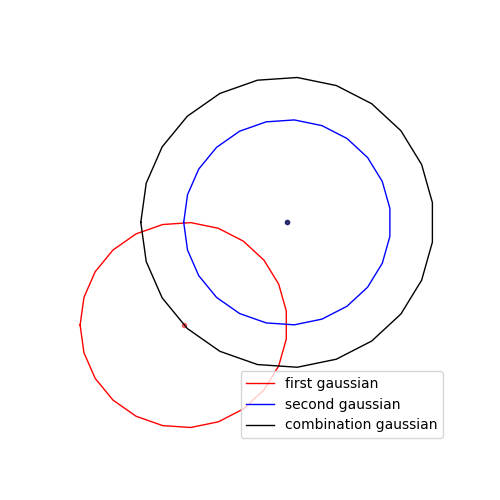##### 2. Fitting a Gaussian distribution to demonstrations

Given a dataset X with \bm{X} \in \mathcal{R}^{2\times\mathrm{nb\_data}}, implement a function fit_gaussian that fits a Gaussian distribution onto this dataset and returns its mean and covariance.

def fit_gaussian(X): mu = np.zeros(2) # implement here sigma = np.eye(2) # implement here return mu, sigma
def fit_gaussian(X): mu = np.mean(X, axis=-1) sigma = (X-mu[:,None]).dot((X-mu[:,None]).T) / nb_data return mu, sigma

Verify your function by plotting the resulting Gaussian distribution along with the dataset. To do this, you can run the code below by changing the noise level. You can also click on the button below to verify your answer (the answer is generated with noise_scale=0.01).

noise_scale = 0.05 # change this to the noise level you want nb_data = 100 x1 = np.linspace(0,1,nb_data) y1 = 0.3*x1 + 0.1 + np.random.randn(nb_data)*noise_scale X1 = np.stack([x1,y1]) mu1, sigma1 = fit_gaussian(X1) plt.close('all') fig,ax = plt.subplots(figsize=(5,5)) plot_gaussian(mu1, sigma1, ax=ax) ax.plot(x1,y1, '.') ax.set_xlabel('x') ax.set_ylabel('y') fig.tight_layout() fig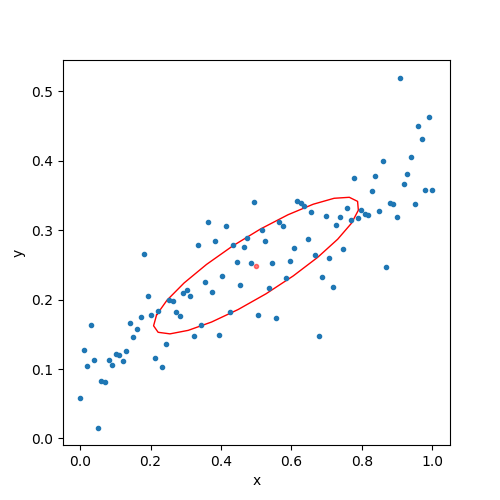##### 3. Intersection or union?

We are given two different sets of demonstration data, each represented by a Gaussian distribution. Depending on the application, we may be interested only in capturing the common parts in both demonstrations, which we will call intersection, or capturing both demonstration datasets together, which we will call union. Intersection and union correspond to the functions that we implemented in this session. Which ones do you think fit to these specifications?

# Create a new dataset X2 x2 = np.linspace(0,1,nb_data) y2 = 1.5*x2 + np.random.randn(nb_data)*noise_scale X2 = np.stack([x2,y2]) # Fit another Gaussian distribution mu2, sigma2 = fit_gaussian(X2) # Compute the resulting Gaussian for the intersection case mu_intersection, sigma_intersection = np.zeros(2), np.eye(2) # implement here # Compute the resulting Gaussian for the union case mu_union, sigma_union = np.zeros(2), np.eye(2) # implement here
# Create a new dataset X2 x2 = np.linspace(0,1,nb_data) y2 = 1.5*x2 + np.random.randn(nb_data)*noise_scale X2 = np.stack([x2,y2]) # Fit another Gaussian distribution mu2, sigma2 = fit_gaussian(X2) # Compute the resulting Gaussian for the intersection case mu_intersection, sigma_intersection, c = product_gaussians(mu1, mu2, sigma1, sigma2) # implement here # Compute the resulting Gaussian for the union case mu_union, sigma_union = fit_gaussian(np.concatenate([X1, X2], axis=-1)) # implement here

Plot these Gaussian distributions to verify your results with the button below.

plt.close('all') fig,ax = plt.subplots(figsize=(5,5)) plot_gaussian(mu2, sigma2, ax, color='b', label='2nd demo') plot_gaussian(mu1, sigma1, ax, color='orange', label='1st demo') plot_gaussian(mu_intersection, sigma_intersection, ax, color='k', label='intersection') plot_gaussian(mu_union, sigma_union, ax, color='g', label='union') ax.legend() ax.plot(x2,y2, '.') ax.plot(x1,y1, '.') ax.set_xlabel('x') ax.set_ylabel('y') fig.tight_layout() fig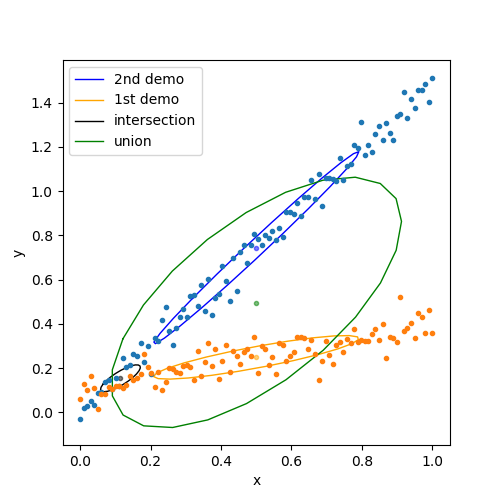Now consider the case where our coordinate system is rotated \pi/3 radians counterclockwise, which also rotated our dataset. Instead of refitting a distribution onto this new rotated dataset, we would like to reuse the mu_union, sigma_union and mu_intersection, sigma_intersection and the rotation matrix R to encode our new rotated dataset.

One function implemented in this section can accomplish this. Call this function with the appropriate arguments and plot the results. You can verify your answer with the button below the code (that you can also use as a hint).

angle = np.pi/3 R = np.array([[np.cos(angle), -np.sin(angle)], [np.sin(angle), np.cos(angle)]]) # Find mu_rotated and sigma_rotated without refitting. mu_intersection_rotated, sigma_intersection_rotated = np.zeros(2), np.eye(2) #implement here mu_union_rotated, sigma_union_rotated = np.zeros(2), np.eye(2) #implement here # Plotting plt.close('all') fig,ax = plt.subplots(figsize=(5,5)) plot_gaussian(mu_intersection, sigma_intersection, ax=ax, color='k', label='intersection') plot_gaussian(mu_intersection_rotated, sigma_intersection_rotated, ax=ax, color='gray', label='intersection rotated') plot_gaussian(mu_union, sigma_union, ax, color='g', label='union') plot_gaussian(mu_union_rotated, sigma_union_rotated, ax=ax, color='g', alpha=0.7, label='union rotated') X2_rotated = R @ X2 X1_rotated = R @ X1 ax.plot(x2,y2, 'r.', alpha=0.1) ax.plot(x1,y1, 'b.', alpha=0.1) ax.plot(X2_rotated,X2_rotated, 'r.', alpha=0.1) ax.plot(X1_rotated,X1_rotated, 'b.', alpha=0.1) ax.set_xlabel('x') ax.set_ylabel('y') ax.legend() fig.tight_layout() fig
angle = np.pi/3 R = np.array([[np.cos(angle), -np.sin(angle)], [np.sin(angle), np.cos(angle)]]) # Find mu_rotated and sigma_rotated without refitting. mu_intersection_rotated, sigma_intersection_rotated = linear_combination(R, R*0, 0, mu_intersection, mu_intersection, sigma_intersection, sigma_intersection) #implement here mu_union_rotated, sigma_union_rotated = linear_combination(R, R*0, 0, mu_union, mu_union, sigma_union, sigma_union) #implement here # Plotting plt.close('all') fig,ax = plt.subplots(figsize=(5,5)) plot_gaussian(mu_intersection, sigma_intersection, ax=ax, color='k', label='intersection') plot_gaussian(mu_intersection_rotated, sigma_intersection_rotated, ax=ax, color='gray', label='intersection rotated') plot_gaussian(mu_union, sigma_union, ax, color='g', label='union') plot_gaussian(mu_union_rotated, sigma_union_rotated, ax=ax, color='g', alpha=0.7, label='union rotated') X2_rotated = R @ X2 X1_rotated = R @ X1 ax.plot(x2,y2, 'r.', alpha=0.1) ax.plot(x1,y1, 'b.', alpha=0.1) ax.plot(X2_rotated,X2_rotated, 'r.', alpha=0.1) ax.plot(X1_rotated,X1_rotated, 'b.', alpha=0.1) ax.set_xlabel('x') ax.set_ylabel('y') ax.legend() fig.tight_layout() fig